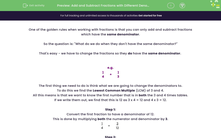# Add and Subtract Fractions with Different Denominators

In this worksheet, students will add and subtract fractions which have different denominators by first finding a common denominator.Key stage:  KS 4

Year:  GCSE

GCSE Subjects:   Maths

GCSE Boards:   Pearson Edexcel, OCR, Eduqas, AQA,

Curriculum topic:   Number, Fractions, Decimals and Percentages

Curriculum subtopic:   Structure and Calculation Fractions

Difficulty level:#### Worksheet Overview

One of the golden rules when working with fractions is that you can only add and subtract fractions which have the same denominator.

So the question is: "What do we do when they don't have the same denominator?"

That's easy - we have to change the fractions so they do have the same denominator

e.g.

 1 4
+
 1 3

The first thing we need to do is think what we are going to change the denominators to.

To do this we find the Lowest Common Multiple (LCM) of 3 and 4.

All this means is that we want to know the first number that is in both the 3 and 4 times tables.

If we write them out, we find that this is 12 as 3 x 4 = 12 and 4 x 3 = 12.

Step 1:

Convert the first fraction to have a denominator of 12.

This is done by multiplying both the numerator and denominator by 3.

 1 4
=
 3 12

Step 2:

Convert the second fraction to have a denominator of 12.

This is done by multiplying both the numerator and denominator by 4.

 1 3
=
 4 12

Step 3:

We can now rewrite our sum with our new fractions, then work it out as a normal addition with like denominators.

Our rule when adding fractions with the same denominator, is to 'add the top row and keep the bottom the same', like this:

 1 4
+
 1 3
=
 3 12
+
 4 12
=
 7 12

Step 4:

Don't forget to simplify your answer into its simplest form if you can.

In this case, 7/12 cannot be simplified any further as 7 and 12 do not have any factors in common, except for 1.

In this activity, you will practise adding and subtracting fractions with different denominators by finding the Lowest Common Multiple (LCM) they have in common and converting them to have the same denominator.

### What is EdPlace?

We're your National Curriculum aligned online education content provider helping each child succeed in English, maths and science from year 1 to GCSE. With an EdPlace account you’ll be able to track and measure progress, helping each child achieve their best. We build confidence and attainment by personalising each child’s learning at a level that suits them.

Get started